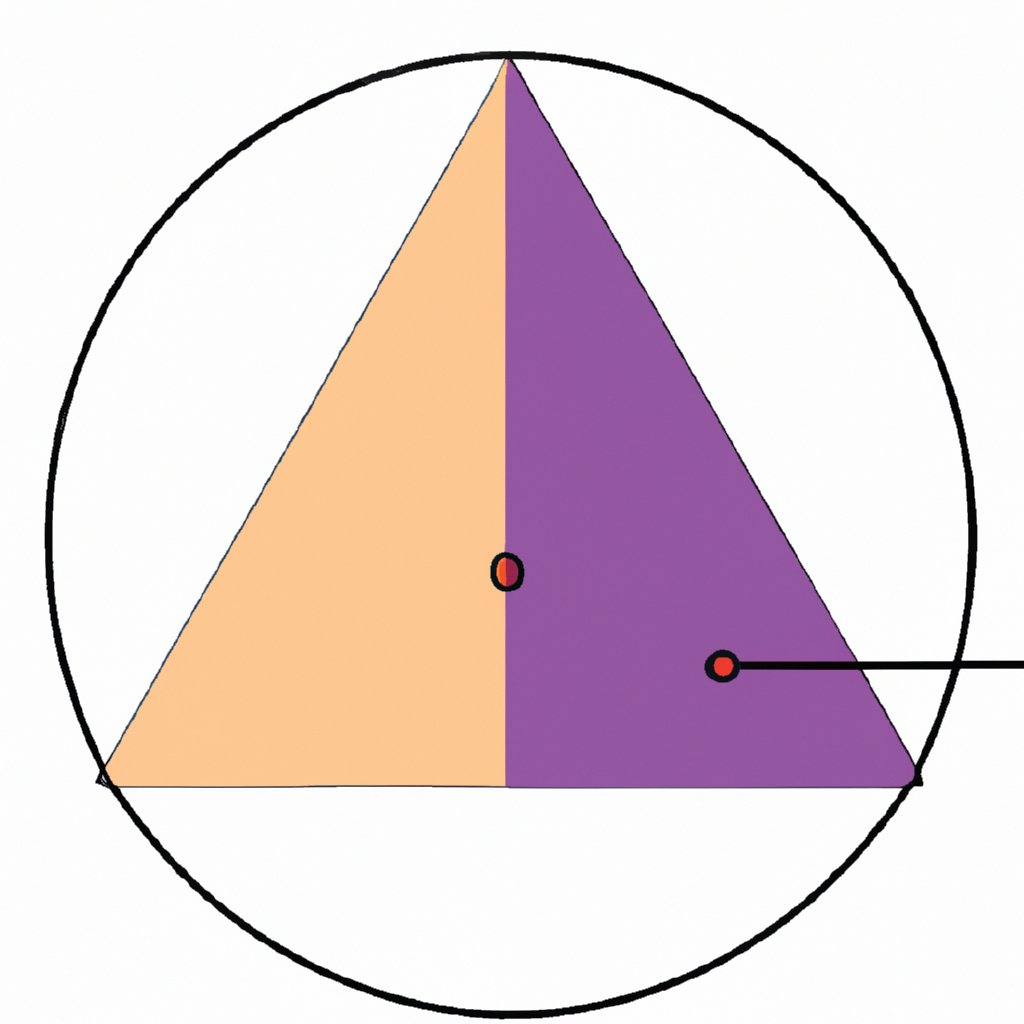## Orthocenter of Triangle Calculator with Steps

Excellent
257
Great Job!
122
Awesome
81
69
Need Help
5
Weary
9

Orthocenter of a triangle is the intersecting point for all the altitudes of the triangle. The point where the altitudes of meet is called the Orthocenter. For instance, if the vertices of triangle ABC are A (-5, 4), B (1, 3), and C (8, 2). The first thing to do is determine the slope of side AB, follow by the slope of the line perpendicular to AB. Repeat the same procedure for side BC to find the slope of the line perpendicular to BC. Apply the equations of a line (point slope) to generate two distinct linear equations with corresponding variables x and y. The unknown values of x and y is the orthocenter of a triangle.

### How The Calculator Works

#### Using The Calculator

• Enter values for each vertex point of triangle, assuming the points are A, B and C
• The first two points is represent the vertex A(x1, x1), the middle block points is the vertex B(x2, y2) and the last block vertex C(x3, y3)
• Hit the equal orange button to compute the worksheet that calculate Orthocenter.

#### Inside the Calculator’s Brain

• The slope of of any two sides of triangles are chosen and calculated using the formula below, for instance, side AB and BC were chosen to illustrate the brain of this calculator• The calculator finds the slope of the altitude  perpendicular to slope of the two chosen sides of triangle• Apply the slope-point form equation as a straight line to generate a new set of linear equations of two variables “x” and “y” using the formula below for the two chosen sides of the triangle.Solve the system of equations of two unknown by method of elimination and resolve for x and y variables respectively.

### Orthocenter Basics• The orthocenter of a triangle is the point of intersection of the three altitudes of the triangle.
• It is an important concept in geometry and can be used to study various properties of triangles.
• The orthocenter can be used to calculate angles, side lengths, and other properties related to the triangle.
• It can also be used to determine whether a given triangle is an acute, obtuse, or right triangle.
• Furthermore, it can help us identify special types of triangles such as equilateral and isosceles triangles.
• This calculator solves various applications such as finding angles and side lengths or determining whether a given triangle has any special properties.Mathcrave Equation Solver provides mathematical learning tools to help students establish a concrete understanding of problem-solving from grade school to university level for free.

## Resources

© 2023 · Mathcrave· Free Step by Step Math Solver.